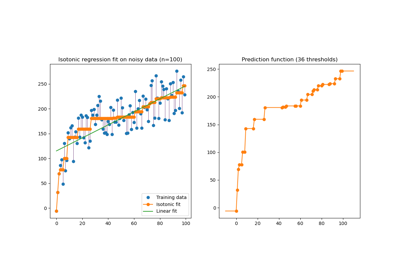# sklearn.isotonic.IsotonicRegression¶

class sklearn.isotonic.IsotonicRegression(*, y_min=None, y_max=None, increasing=True, out_of_bounds='nan')[source]

Isotonic regression model.

Read more in the User Guide.

New in version 0.13.

Parameters:
y_minfloat, default=None

Lower bound on the lowest predicted value (the minimum value may still be higher). If not set, defaults to -inf.

y_maxfloat, default=None

Upper bound on the highest predicted value (the maximum may still be lower). If not set, defaults to +inf.

increasingbool or ‘auto’, default=True

Determines whether the predictions should be constrained to increase or decrease with X. ‘auto’ will decide based on the Spearman correlation estimate’s sign.

out_of_bounds{‘nan’, ‘clip’, ‘raise’}, default=’nan’

Handles how X values outside of the training domain are handled during prediction.

• ‘nan’, predictions will be NaN.

• ‘clip’, predictions will be set to the value corresponding to the nearest train interval endpoint.

• ‘raise’, a ValueError is raised.

Attributes:
X_min_float

Minimum value of input array X_ for left bound.

X_max_float

Maximum value of input array X_ for right bound.

X_thresholds_ndarray of shape (n_thresholds,)

Unique ascending X values used to interpolate the y = f(X) monotonic function.

New in version 0.24.

y_thresholds_ndarray of shape (n_thresholds,)

De-duplicated y values suitable to interpolate the y = f(X) monotonic function.

New in version 0.24.

f_function

The stepwise interpolating function that covers the input domain X.

increasing_bool

Inferred value for increasing.

sklearn.linear_model.LinearRegression

Ordinary least squares Linear Regression.

sklearn.ensemble.HistGradientBoostingRegressor

Gradient boosting that is a non-parametric model accepting monotonicity constraints.

isotonic_regression

Function to solve the isotonic regression model.

Notes

Ties are broken using the secondary method from de Leeuw, 1977.

References

Isotonic Median Regression: A Linear Programming Approach Nilotpal Chakravarti Mathematics of Operations Research Vol. 14, No. 2 (May, 1989), pp. 303-308

Isotone Optimization in R : Pool-Adjacent-Violators Algorithm (PAVA) and Active Set Methods de Leeuw, Hornik, Mair Journal of Statistical Software 2009

Correctness of Kruskal’s algorithms for monotone regression with ties de Leeuw, Psychometrica, 1977

Examples

>>> from sklearn.datasets import make_regression
>>> from sklearn.isotonic import IsotonicRegression
>>> X, y = make_regression(n_samples=10, n_features=1, random_state=41)
>>> iso_reg = IsotonicRegression().fit(X, y)
>>> iso_reg.predict([.1, .2])
array([1.8628..., 3.7256...])


Methods

 fit(X, y[, sample_weight]) Fit the model using X, y as training data. fit_transform(X[, y]) Fit to data, then transform it. get_feature_names_out([input_features]) Get output feature names for transformation. get_params([deep]) Get parameters for this estimator. Predict new data by linear interpolation. score(X, y[, sample_weight]) Return the coefficient of determination of the prediction. set_params(**params) Set the parameters of this estimator. Transform new data by linear interpolation.
fit(X, y, sample_weight=None)[source]

Fit the model using X, y as training data.

Parameters:
Xarray-like of shape (n_samples,) or (n_samples, 1)

Training data.

Changed in version 0.24: Also accepts 2d array with 1 feature.

yarray-like of shape (n_samples,)

Training target.

sample_weightarray-like of shape (n_samples,), default=None

Weights. If set to None, all weights will be set to 1 (equal weights).

Returns:
selfobject

Returns an instance of self.

Notes

X is stored for future use, as transform needs X to interpolate new input data.

fit_transform(X, y=None, **fit_params)[source]

Fit to data, then transform it.

Fits transformer to X and y with optional parameters fit_params and returns a transformed version of X.

Parameters:
Xarray-like of shape (n_samples, n_features)

Input samples.

yarray-like of shape (n_samples,) or (n_samples, n_outputs), default=None

Target values (None for unsupervised transformations).

**fit_paramsdict

Returns:
X_newndarray array of shape (n_samples, n_features_new)

Transformed array.

get_feature_names_out(input_features=None)[source]

Get output feature names for transformation.

Parameters:
input_featuresarray-like of str or None, default=None

Ignored.

Returns:
feature_names_outndarray of str objects

An ndarray with one string i.e. [“isotonicregression0”].

get_params(deep=True)[source]

Get parameters for this estimator.

Parameters:
deepbool, default=True

If True, will return the parameters for this estimator and contained subobjects that are estimators.

Returns:
paramsdict

Parameter names mapped to their values.

predict(T)[source]

Predict new data by linear interpolation.

Parameters:
Tarray-like of shape (n_samples,) or (n_samples, 1)

Data to transform.

Returns:
y_predndarray of shape (n_samples,)

Transformed data.

score(X, y, sample_weight=None)[source]

Return the coefficient of determination of the prediction.

The coefficient of determination $$R^2$$ is defined as $$(1 - \frac{u}{v})$$, where $$u$$ is the residual sum of squares ((y_true - y_pred)** 2).sum() and $$v$$ is the total sum of squares ((y_true - y_true.mean()) ** 2).sum(). The best possible score is 1.0 and it can be negative (because the model can be arbitrarily worse). A constant model that always predicts the expected value of y, disregarding the input features, would get a $$R^2$$ score of 0.0.

Parameters:
Xarray-like of shape (n_samples, n_features)

Test samples. For some estimators this may be a precomputed kernel matrix or a list of generic objects instead with shape (n_samples, n_samples_fitted), where n_samples_fitted is the number of samples used in the fitting for the estimator.

yarray-like of shape (n_samples,) or (n_samples, n_outputs)

True values for X.

sample_weightarray-like of shape (n_samples,), default=None

Sample weights.

Returns:
scorefloat

$$R^2$$ of self.predict(X) wrt. y.

Notes

The $$R^2$$ score used when calling score on a regressor uses multioutput='uniform_average' from version 0.23 to keep consistent with default value of r2_score. This influences the score method of all the multioutput regressors (except for MultiOutputRegressor).

set_params(**params)[source]

Set the parameters of this estimator.

The method works on simple estimators as well as on nested objects (such as Pipeline). The latter have parameters of the form <component>__<parameter> so that it’s possible to update each component of a nested object.

Parameters:
**paramsdict

Estimator parameters.

Returns:
selfestimator instance

Estimator instance.

transform(T)[source]

Transform new data by linear interpolation.

Parameters:
Tarray-like of shape (n_samples,) or (n_samples, 1)

Data to transform.

Changed in version 0.24: Also accepts 2d array with 1 feature.

Returns:
y_predndarray of shape (n_samples,)

The transformed data.

## Examples using sklearn.isotonic.IsotonicRegression¶Isotonic Regression

Isotonic Regression